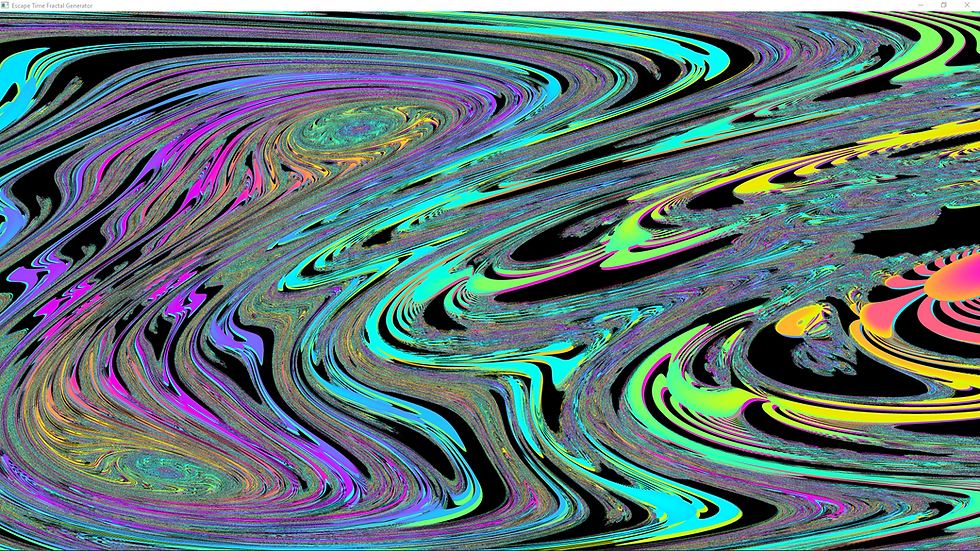top of page

# Progress made on my fractal program part two: create your own fractals!

This took around a week to do but it was well worth it. Clicking the "custom function" checkbox will take you to this UI:The proper way to do this would have been with a graph system but that was outside the scope of this project (and would have taken much longer than a week), so I am using a less user friendly "list of commands" system. I did my best to polish it up as much as I could though.

For the starting function, if you want a mandelbrot-like set, start with a constant and "move" it to your command output for the starting function. For the recursive function, multiply (n-1) by itself and add the result of that command to the input position:For the julia set, you would "move" the input position instead of a constant in your starting function, and end your recursive function by adding a constant:At this point you can just start doing whatever you want. Just throw random math together and you'll usually get something interesting:If you want to tweak one of your constants, select it, hold V, and mouse over the rendering window. It will set a value to the point you're mousing over.

You might be surprised by what you stumble upon just messing around:if you get something like this, lower the "Upsample" value to around 0.2 to speed up the rendering, zoom in on an interesting part of the fractal, and then raise the iterations to a higher value and the upsample value to 2 or 4 for a high quality image:Remember, you can swap out the color ramp without re-rendering the fractal if you want a different color scheme.Tetration fractal / "power tower" fractal (n = input^(n-1))n = tan(n-1)^2n = (n-1)^ln(n-1)n = cosh(n-1)